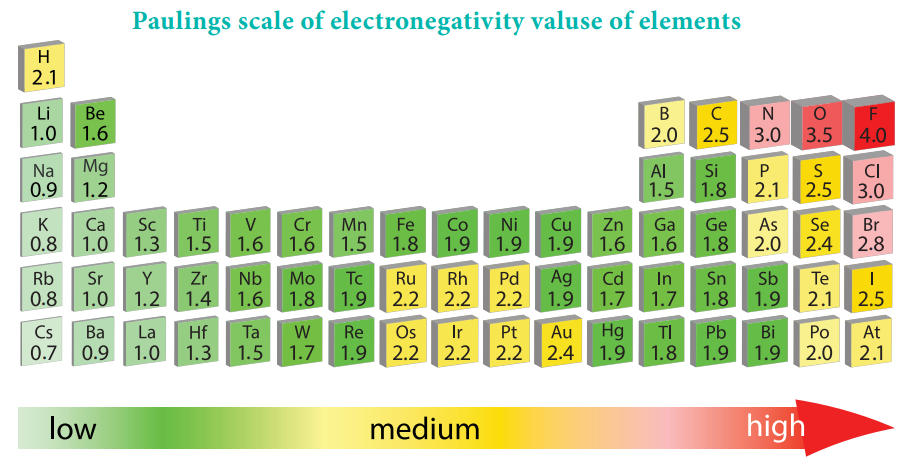Home | | Chemistry 11th std | Electronegativity

# ElectronegativityIt is defined as the relative tendency of an element present in a covalently bonded molecule, to attract the shared pair of electrons towards itself.

Electronegativity:

It is defined as the relative tendency of an element present in a covalently bonded molecule, to attract the shared pair of electrons towards itself.

Electronegativity is not a measurable quantity. However, a number of scales are available to calculate its value. One such method was developed by Pauling, he assigned arbitrary value of electronegativities for hydrogen and fluorine as 2.2 and 4.0 respectively. Based on this the electronegativity values for other elements can be calculated using the following expression

A – χB ) = 0.182 √EAB – (EAA*EBB)½

Where EAB, EAA and EBB are the bond dissociation energies of AB, A2 and B2 molecules respectively.

The electronegativity of any given element is not a constant and its value depends on the element to which it is covalently bound. The electronegativity values play an important role in predicting the nature of the bond.

## Variation of Electronegativity in a period:

The electronegativity generally increases across a period from left to right. As discussed earlier, the atomic radius decreases in a period, as the attraction between the valence electron and the nucleus increases. Hence the tendency to attract shared pair of electrons increases. Therefore, electronegativity also increases in a period## Variation of Electronegativity in a group:

The electronegativity generally decreases down a group. As we move down a group the atomic radius increases and the nuclear attractive force on the valence electron decreases. Hence, the electronegativity decreases.

Noble gases are assigned zero electronegativity. The electronegativity values of the elements of s-block show the expected decreasing order in a group. Except 13th and 14th group all other p-block elements follow the expected decreasing trend in electronegativity.Study Material, Lecturing Notes, Assignment, Reference, Wiki description explanation, brief detail
11th Chemistry : UNIT 3 : Periodic Classification of Elements : Electronegativity |